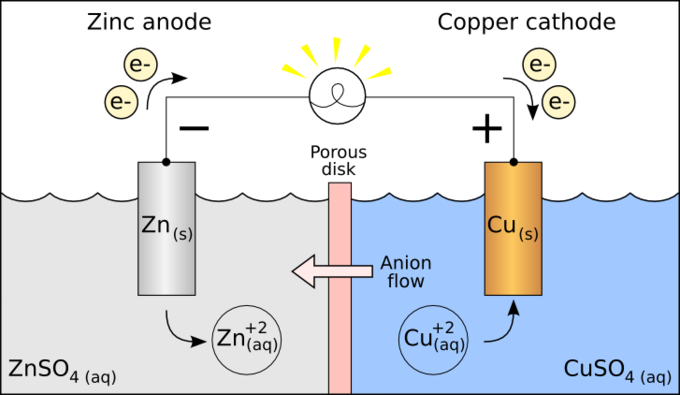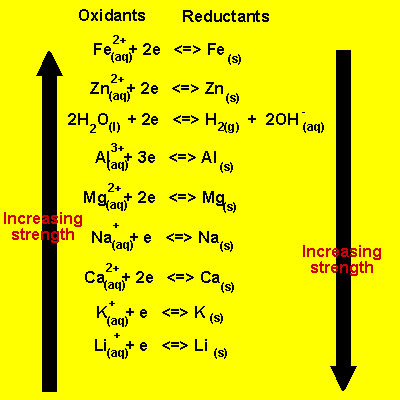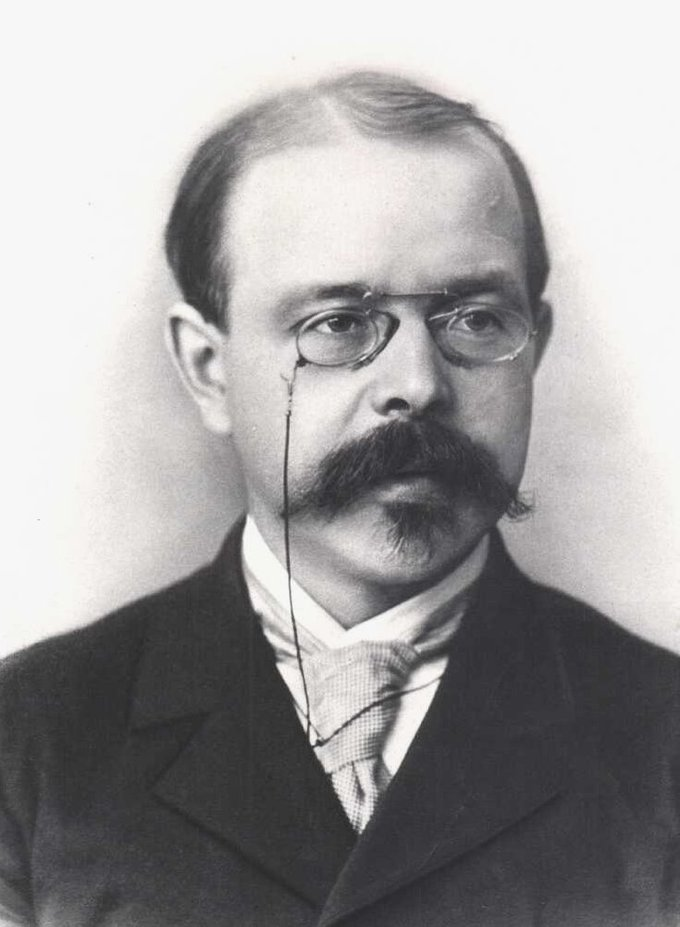## Standard Reduction Potentials

Standard reduction potentials provide a systematic measurement for different molecules’ tendency to be reduced.

### Learning Objectives

Recall that a positive reduction potential indicates a thermodynamically favorable reaction relative to the reduction of a proton to hydrogen

### Key Takeaways

#### Key Points

• A reduction potential measures the tendency of a molecule to be reduced by taking up new electrons.
• The standard reduction potential is the reduction potential of a molecule under specific, standard conditions.
• Standard reduction potentials can be useful in determining the directionality of a reaction.
• The reduction potential of a given species can be considered to be the negative of the oxidation potential.

#### Key Terms

• reduce: To add electrons/hydrogen or to remove oxygen.
• standard hydrogen electrode: A redox electrode which forms the basis of the thermodynamic scale of oxidation-reduction potentials; used as a standard against which other electrodes are measured.

### Reduction Potential

Reduction potential (also known as redox potential, oxidation/reduction potential, or Eh) measures the tendency of a chemical species to acquire electrons and thereby be reduced. Reduction potential is measured in volts (V) or millivolts (mV). Each species has its own intrinsic reduction potential. The more positive the potential, the greater the species’ affinity for electrons, or the more the species tends to be reduced.Oxidation-reduction in a galvanic cell: In this galvanic cell, zinc reduces copper cations. The reaction yields zinc cations and neutral copper metal.

The standard reduction potential (E0) is measured under standard conditions:

• 25 °C
• 1 M concentration for each ion participating in the reaction
• Partial pressure of 1 atm for each gas that is part of the reaction
• Metals in their pure states

### Standard Reduction Potential

The standard reduction potential is defined relative to a standard hydrogen electrode (SHE) reference electrode, which is arbitrarily given a potential of 0.00 volts. The values below in parentheses are standard reduction potentials for half-reactions measured at 25 °C, 1 atmosphere, and with a pH of 7 in aqueous solution.

• CH3COOH + 2H+ + 2e → CH3CHO + H2O (-0.58)
• 2H+ + 2 e → H2 (0.0)
• O2 + 2H+ + 2e → H2O2 (+0.7)
• O2 + 4H+ + 4e → 2H2O (+1.64)

Since the reduction potential measures the intrinsic tendency for a species to undergo reduction, comparing standard reduction potential for two processes can be useful for determining how a reaction will proceed.

Historically, many countries, including the United States and Canada, used standard oxidation potentials rather than reduction potentials in their calculations. These are simply the negative of standard reduction potentials, so it is not a difficult conversion in practice. However, because these can also be referred to as “redox potentials,” the terms “reduction potentials” and “oxidation potentials” are preferred by the IUPAC. The two may be explicitly distinguished by using the symbol E0r for reduction and E0o for oxidation.

## Predicting Spontaneous Direction of a Redox Reaction

The direction of a redox reaction depends on the relative strengths of the oxidants and reductants in a solution.

### Learning Objectives

Predict the direction of electron flow in a redox reaction given the reduction potentials of the two half-reactions

### Key Takeaways

#### Key Points

• Sometimes, the direction of a redox reaction can be determined by estimating the relative strengths of the reductants and oxidants.
• In situations where an electrochemical series is not sufficient to absolutely determine the direction of a redox reaction, the standard electrode potential, Eo, can be used.
• A negative value of cell potential indicates a reducing environment, while a positive value indicates an oxidizing environment.

#### Key Terms

• standard electrode potential: An electrode potential measured under standard conditions (298 K, 1 atm, and 1 M).

### Predicting the Redox Half-Reactions

Generally, the direction of a redox reaction depends on the relative strengths of oxidants and reductants in a solution. In simple situations, an electrochemical series can be very useful for determining the direction of the reaction.Electrochemical series: In order to predict if two reactants will take part in a spontaneous redox reaction, it is important to know how they rank in an electrochemical series.

In the table provided, the most easily reduced element is Li and the most easily oxidized is iron. This means that Li would be written as the reduction half-reaction when compared to any other element in this table. On the other hand, Fe would be written as the oxidation half-reaction when compared to any other element on this table.

Some reactions cannot be “eyeballed” in this manner. These reactions require a more mathematical method to determine the direction. To figure this out, it is important to consider the standard electrode potential, which is a measure of the driving force behind a reaction. The sign of the standard electrode potential indicates in which direction the reaction must proceed in order to achieve equilibrium.

For example, let’s look at the reaction between zinc and acid:

$\text{Zn}(\text{s}) + 2 \text{H}^+(\text{aq}) \leftrightarrow \text{Zn}^{2+}(\text{aq}) + \text{H}_2(\text{g})$

Oxidation: $\text{Zn}(\text{s}) \rightarrow \text{Zn}^{2+}(\text{aq}) + 2\text{e}^- \ \text{E}^\text{o} = 0.76\ \text{V}$

Reduction: $2\text{H}^+ + 2\text{e}^- \rightarrow \text{H}_2 \ \text{E}^\text{o} = 0.00\ V$

Eo = 0.76 V

The positive Eo value indicates that at STP this reaction must proceed to the right in order to achieve equilibrium. This is to say, a positive Eo value indicates a reaction has equilibrium constants that favor the products.

What happens to the standard electrode potential when the reaction is written in the reverse direction? Neither the relative strengths of the oxidizing or reducing agents nor the magnitude of the potential will change. However, what will change is the sign of the standard electrode potential. This means we can convert a spontaneous reaction to an unfavorable one and vice versa. For example if we turn the zinc oxidation half-reaction around ($\text{Zn}^{2+} + 2\text{e}^- \rightarrow \text{Zn} \ \text{E}^\text{o} = -0.76 \text{V}$), the cell potential is reversed.

The relative reactivities of different half-reactions can be compared to predict the direction of electron flow. Half-reaction equations can be combined if one is reversed to oxidation in a manner that cancels out the electrons.

## Predicting if a Metal Will Dissolve in Acid

A metal is soluble in acid if it displaces H2 from solution, which is determined by the metal’s standard reduction potential.

### Learning Objectives

Predict whether a metal will dissolve in acid, given its reduction potential

### Key Takeaways

#### Key Points

• Some metals have stronger “replacing” power than others, indicating that they are more likely to reduce.
• Although H2 is not a metal, it can still be “replaced” by some strongly reducing metals.
• The tendency of a metal to “displace” hydrogen gas from acidic solution determines its solubility; if the metal cannot displace hydrogen, it will not be oxidized and will remain insoluble.
• You can determine if a metal will dissolve in acid by comparing the standard reduction potential of the metal to that of hydrogen gas.

#### Key Terms

• reduce: To add electrons/hydrogen or to remove oxygen.
• oxidize: To increase the valence (the positive charge) of an element by removing electrons.

### Activity of Metals

Some metals can be considered to be more “active” than others, in the sense that a more active metal can replace a less active one from a solution of its salt. The classic example is of zinc displacing copper:

$\text{Zn} (\text{s}) + \text{Cu}^{2+} \rightarrow \text{Zn}^{2+} + \text{Cu} (\text{s})$

Here, zinc is more active than copper because it can replace copper in solution. If you immerse a piece of metallic zinc in a solution of copper sulfate, the surface of the zinc quickly becomes covered with a coating of finely divided copper. The blue color of the solution diminishes as copper(II) ion is being replaced.

Similar comparisons of other metals have made it possible to arrange them in order of their increasing electron -donating, or reducing, power. This sequence is known as the electromotive, or activity, series of the metals.

• Activity level 1 (highest): Li, K, Ca, Na
• Activity level 2: Mg, Al, Mn, Zn, Fe
• Activity level 3: Ni, Sn, Pb
• Activity level 4 (lowest): Cu, Ag, Pt, Au

### Activity Series

The activity series has long been used to predict the direction of oxidation -reduction reactions. Consider, for example, the oxidation of copper by metallic zinc mentioned above. Zinc is near the top of the activity series, meaning that this metal has a strong tendency to lose electrons. Copper, on the other hand, is a poorer electron donor, and therefore its oxidized form, Cu, is a fairly good electron acceptor. We would therefore expect the following reaction to proceed in the direction already indicated, rather than in the reverse direction:

$\text{Zn} (\text{s}) + \text{Cu}^{2+} \rightarrow \text{Zn}^{2+} + \text{Cu} (\text{s})$

An old-fashioned way of expressing this is to say that “zinc will replace copper from solution.”

Note that the table also takes the replacement of hydrogen (H2) into account. Although H2 is not a metal, it can still be “replaced” by some strongly reducing metals. The tendency of a metal to “replace” hydrogen gas from acidic solution will determine its solubility in that solution.

### Reduction Potentials

Each half-cell is associated with a potential difference whose magnitude depends on the nature of the particular electrode reaction and on the concentrations of the dissolved species. The sign of this potential difference depends on the direction (oxidation or reduction) in which the electrode reaction proceeds. In order to express them in a uniform way, we follow the rule that half-cell potentials are always defined for the reduction direction. Therefore, the half-cell potential for the Zn/Zn2+ electrode always refers to the reduction reaction:

$\text{Zn}^{2+} + 2\text{e}^ - \rightarrow \text{Zn} (\text{s})$

In the cell Zn (s) | Zn2+ (aq) || Cu2+ (aq) | Cu (s), the zinc appears on the left side, indicating that it is being oxidized, not reduced. For this reason, the potential difference contributed by the left half-cell has the opposite sign to its conventional reduction half-cell potential. These values can be determined using standard reduction potentials, which can often be looked up. Using the standard reduction potentials of a reaction, one can determine how likely a given metal is to accept or donate electrons. For H2, you can quantitatively deduce whether the given metal will dissolve in aqueous solution.

### Example

Will Zinc metal readily dissolve when added to HCl?

The net ionic equation for dissolving Zn in HCl would look like this:

$\text{Zn} + 2\text{H}^+ \rightarrow \text{Zn}^{2+} + \text{H}_2$

Set up the oxidation and reduction half-reactions with their cell potential:

$\text{Zn} \rightarrow \text{Zn}^{2+} + 2\text{e}^- \ \ \ \text{E}^\text{o} = 0.76 \ \text{V}$

$2\text{H}^+ + 2\text{e}^- \rightarrow \text{H}_2 \ \ \ \text{E}^\text{o} = 0.00\ V$

Adding the two half-reactions together gives the overall equation and a positive value for E0. This means the reaction is spontaneous and Zn will dissolve in HCl.

## Thermodynamics of Redox Reactions

The thermodynamics of redox reactions can be determined using their standard reduction potentials and the Nernst equation.

### Learning Objectives

Translate between the equilibrium constant/reaction quotient, the standard reduction potential, and the Gibbs free energy change for a given redox reaction

### Key Takeaways

#### Key Points

• Redox reactions are reactions that transfer electrons between species.
• The Nernst equation allows us to calculate the reduction potential of a redox reaction under “non-standard” conditions.
• When the system is at equilibrium, the Nernst equation can be simplified and written in terms of the equilibrium constant, K, which, in turn, allows for derivation of an equation to determine the change in Gibbs free energy ( $\Delta \text{G}$ ) of the reaction.

#### Key Terms

• Gibbs free energy: A thermodynamic potential that measures the “useful” or process-initiating work obtainable from a thermodynamic system at a constant temperature and pressure.
• standard reduction potential: The reduction potential of a reaction measured under standard conditions: 25 °C, a 1 M concentration for each participating ion, a partial pressure of 1 atm for each gas, and metals in pure states.

### Reduction Potential

In order to calculate thermodynamic quantities like change in Gibbs free energy $\Delta \text{G}$ for a general redox reaction, an equation called the Nernst equation must be used. Walther Nernst was a German chemist and physicist who developed an equation in the early 20th century to relate reduction potential, temperature, concentration, and moles of electrons transferred.Walther Nernst: A portrait of Walther Nernst.

The Nernst equation allows the reduction potential to be calculated at any temperature and concentration of reactants and products; the standard reaction potential must be measured at 298K and with each solution at 1M.

The Nernst equation is:

$\text{E} = \text{E}^0 - (\frac{\text{RT}}{\text{nF}})\ln \text{Q}$

Where the terms are as follows:

• E is the reduction potential for the specified non-standard state
• E0 is the standard reduction potential
• R and F are the gas and Faraday constants, respectively
• n is the number of electrons transferred in the reaction
• Q is the reaction quotient $\frac{\text{C}^\text{c}\text{D}^\text{d}}{\text{A}^\text{a}\text{B}^\text{b}}$. The uppercase letters are concentrations and the lowercase letters are stoichiometric coefficients for the reaction $\text{aA} + \text{bB} \rightarrow \text{cC} + \text{dD}$

If T is held constant at 298K, the Nernst equation can be condensed using the values for the constants R and F:

$\text{E} = \text{E}^0 - (\frac{0.0257}{\text{n}})\ln \text{Q}$

It can be further simplified if the reaction has reached equilibrium, as in that case Q is the equilibrium constant K:

$\ln\text{ K} = \frac{\text{nE}^0}{0.0257}$

This equation allows the equilibrium constant to be calculated just from the standard reduction potential and the number of electrons transferred in the reaction.

### Gibbs Free Energy Charge

The relationship between the Gibbs free energy change and the standard reaction potential is:

$\Delta \text{G} = -\text{nFE}^0$

In this equation:

• $\Delta \text{G}$ is the change in free energy
• n is the number of moles
• E0 is the standard potential
• F is the Faraday constant